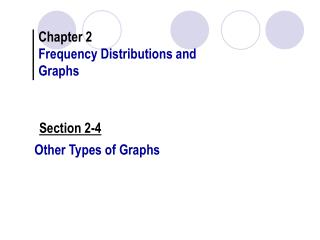DownloadDownload PresentationOther Types of Graphs

# Other Types of Graphs

Télécharger la présentation## Other Types of Graphs

- - - - - - - - - - - - - - - - - - - - - - - - - - - E N D - - - - - - - - - - - - - - - - - - - - - - - - - - -
##### Presentation Transcript

1. Chapter 2 Frequency Distributions and Graphs Section 2-4 Other Types of Graphs

2. Suggestions for Drawing Pareto Charts 1. Make the bars the same width. 2. Arrange the data from largest to smallest according to frequency. 3. Make the units that are used for the frequency equal in size.

3. Section 2-4 Exercise #1 The population of federal prisons, according to the most serious offenses, consists of the following. Make a Pareto chart of the population.

4. A time series graph represents data that occur over a specific period of time. • A pie graph is a circle that is divided into sections or wedges according to the percentage of frequencies in each category of the distribution.

5. Section 2-4 Exercise #7 The data represent the personal consumption (in billions of dollars) for tobacco in the United States. Draw a time series graph for the data and explain the trend.

6. Section 2-4 Exercise #11 The assets of the richest 1% of Americans are distributed as follows. Make a pie chart for the percentages.

7. Stem and Leaf Plots • A stem and leaf plot is a data plot that uses part of the data value as the stem and part of the data value as the leaf to form groups or classes.

8. Section 2-4 Exercise #15 The age at inauguration for each U.S. President is shown below. Construct a stem and leaf plot and analyze the data.

9. Chapter 2: Frequency Distributions and Graphs Section 2.5Paired Data and Scatter Plots

10. Scatter Plots • A scatter plot is a graph of order pairs of data values that is used to determine if a relationship exists between the two variables.

11. Analyzing the Scatter Plot 1. A positive linear relationship exists when the points fall approximately in an ascending straight line and both the x and y values increase at the same time. The relationship then is that as the values for the x variable increase, the values for the y variable are increasing. 2. A negative linear relationship exists when the points fall approximately in a descending straight line from left to right. The relationship then is that as the x values are increasing, the y values are decreasing, or vice versa. 3. A nonlinear relationship exists when the points fall in a curved line. The relationship is described by the nature of the curve. 4. No relationship exists when there is no discernable pattern of the points.

12. Section 2-5 Exercise #5 100 80 60 40 20 0 No. of Stories 0 200 400 600 800 1000 Height The data represent the heights in feet and the number of stories of the tallest buildings in Pittsburgh. Draw a scatter plot for the data and describe the relationship.

13. Section 2-5 Exercise #7 A study was conducted to determine if the amount a person spends per month on recreation is related to the person’s income.Draw a scatter plot and comment on the nature of the relationship. 250 220 180 150 120 90 60 30 Amount Spent on Recreation 700 800 900 1000 1100 1200 1300 Monthly Income

14. Section 2-5 Exercise #9 A statistics instructor wishes to determine if a relationship exists between the final exam score in Statistics 101 and the final exam scores of the same students who took Statistics 102. Draw a scatter plot and comment on the nature of the relationship. 100 90 80 70 60 Final Exam Score in Stat 102 60 70 80 90 100 Final Exam Score in Stat 101

15. Section 2-5 Exercise #11 1000 750 500 250 The data shown indicate the number of tournaments and the earnings in thousands of dollars of 10 randomly selected LPGA golfers. Draw a scatter plot for the data and determine the nature of the relationship. Earnings 15 20 25 30 35 Number of Tournaments

16. No. of absences, x 10 12 2 0 8 5 70 65 96 94 75 82 Final Grade, y Section 2-5 Exercise #13 An educator wants to see if there is a relationship between the number of absences a student has and his or her final grade in a course.Draw a scatter plot and comment on the nature of the relationship. 100 90 80 70 60 Final Grade 0 5 10 15 Number of Absences